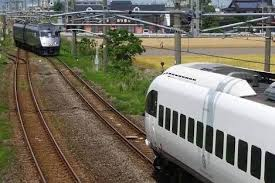# Trains Meeting!

Algebra Level 2

Two stations A and B are $110~ km$ apart on a straight line. One train starts from A at $8:00$ AM and travels towards $B$ at $40~ km/h$. Another train starts from B at $10:00$ AM and travels towards $A$ at $50~ km/h$. At what time will the both trains meet?

• Assume that both the trains are traveling with constant speed throughout the journey.×